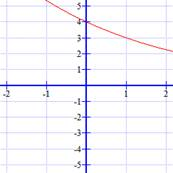Sample Problem

Decide whether the graph below is an example of exponential growth or decay, then write a rule for itSolution

We can see from the graph that this is an example of exponential decay, meaning choice A and C are not correct.

We know that the value must be 4 because when x is 0, y is 4, so y = 4 × 0.75x

Choice D is correct.